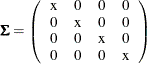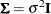### Example 27.5 Testing Some Standard Covariance Pattern Hypotheses

In Example 27.3, you test the uncorrelatedness of variables by using the MSTRUCT model specification. In Example 27.4, you test the sphericity of the covariance matrix by using the same model specification technique. In both examples, you need to specify the parameters in the covariance structure model explicitly by using the MATRIX statements.

Some covariance patterns are well-known in multivariate statistics, including the two tests in Example 27.3 and Example 27.4. To facilitate the tests of these standard covariance patterns, PROC CALIS provides the COVPATTERN= option to specify those standard covariance patterns more efficiently. With the COVPATTERN=option, you do not need to use the MSTRUCT and MATRIX statements to specify the covariance patterns explicitly. See the COVPATTERN= option for the supported covariance patterns. This example illustrates the use of the COVPATTERN= option.

In Example 27.3, you conduct a test of uncorrelatedness for the four variables in the sales data (see Example 27.1 for the data set). That is, the variables are hypothesized to be uncorrelated and only the four variances on the diagonal of the covariance matrix are population parameters of interest. The null hypothesis for the population covariance matrix iswhere each x represents a distinct parameter (that is, the diagonal elements are not constrained with each other). You can test the diagonal covariance pattern easily by the following specification:

proc calis data=sales covpattern=diag;
run;


The COVPATTERN=DIAG option specifies the required diagonal covariance pattern for the test. PROC CALIS then sets up the covariance structures automatically. Output 27.5.1 shows the initial specification of the covariance pattern. As required, only the diagonal elements are parameters in the test and the other elements are fixed to zero. PROC CALIS names the variance parameters automatically—that is, _varparm_1_varparm_4 are the four parameters for the variances. This is the same pattern as shown in Output 27.3.1 of Example 27.3, although the parameter names are different.

Output 27.5.1: Initial Diagonal Pattern for the Covariance Matrix of the Sales Data

Initial MSTRUCT _COV_ Matrix
q1 q2 q3 q4
q1
 . [_varparm_1]
 0
 0
 0
q2
 0
 . [_varparm_2]
 0
 0
q3
 0
 0
 . [_varparm_3]
 0
q4
 0
 0
 0
 . [_varparm_4]

Output 27.5.2 shows the results of the chi-square test of the diagonal covariance pattern. The chi-square is 5.44 (df = 6, p = 0.4887), which is not significant. You fail to reject the null hypothesis of the diagonal covariance pattern in the population.

Output 27.5.2: Fit Summary of the Diagonal Covariance Pattern Test for the Sales Data

Fit Summary
Chi-Square 5.4400
Chi-Square DF 6
Pr > Chi-Square 0.4887

The numerical results shown in Output 27.5.2 are different from those of the same test by using the MSTRUCT model specification, which is shown in Output 27.3.2 of Example 27.3, although you do not reject the null hypothesis in both cases. The reason is that with the use of COVPATTERN= option, PROC CALIS applies the appropriate chi-square correction to the test statistic automatically. In the current example, the chi-square correction due to Bartlett (1950) has been applied. Test results with chi-square corrections are theoretically more accurate.

To obtain the same numerical results as those in Output 27.3.2, you can turn off the chi-square correction by using the CHICORRECT=0 option, as shown in the following specification:

proc calis data=sales covpattern=diag chicorrect=0;
run;


Output 27.5.3 shows the fit summary results without any chi-square correction. The numerical results match exactly to those shown in Output 27.3.2 of Example 27.3.

Output 27.5.3: Fit Summary of the Diagonal Covariance Pattern Test for the Sales Data: No Chi-Square Correction

Fit Summary
Chi-Square 6.5280
Chi-Square DF 6
Pr > Chi-Square 0.3667

Example 27.4 tests the sphericity of the covariance matrix of the same data set. The null hypothesis for the population covariance matrix iswhereis an unknown positive constant andis an identity matrix. You can use the following specification to test this hypothesis easily:

proc calis data=sales covpattern=sigsqi;
run;


Output 27.5.4 shows the initial specification of the covariance pattern. As required, the diagonal elements are all the same parameter named _varparm, and all the off-diagonal elements are fixed to zero. This is the same pattern as shown in Output 27.4.1 of Example 27.4.

Output 27.5.4: Initial Covariance Pattern for the Sphericity Test on the Sales Data

Initial MSTRUCT _COV_ Matrix
q1 q2 q3 q4
q1
 . [_varparm]
 0
 0
 0
q2
 0
 . [_varparm]
 0
 0
q3
 0
 0
 . [_varparm]
 0
q4
 0
 0
 0
 . [_varparm]

Output 27.5.5 shows the fit summary of the sphericity test. The chi-square is 27.747 (df = 9, p = 0.0011), which is statistically significant. You reject the sphericity hypothesis for the population covariance matrix.

Output 27.5.5: Fit Summary of the Sphericity Test on the Sales Data

Fit Summary
Chi-Square 27.7470
Chi-Square DF 9
Pr > Chi-Square 0.0011

Again, the numerical results in Output 27.5.5 are different from those shown in Output 27.4.2 of Example 27.4. This is because with the COVPATTERN=SIGSQI option, the chi-square correction due to Box (1949) has been applied in the current example. To turn off the automatic chi-square correction, you can use the following specification:

proc calis data=sales covpattern=sigsqi chicorrect=0;
run;


As expected, the numerical results in Output 27.5.6 match exactly to those of in Output 27.4.2 of Example 27.4.

Output 27.5.6: Fit Summary of the Sphericity Test on the Sales Data: No Chi-Square Correction

Fit Summary
Chi-Square 31.5951
Chi-Square DF 9
Pr > Chi-Square 0.0002

This example shows that for the tests of some standard covariance patterns, you can use the COVPATTERN= option directly. As compared with the use of the explicit MSTRUCT model specifications, which are shown in Example 27.3 and Example 27.4, the use of COVPATTERN= option is more efficient and less error-prone in coding. In addition, it can apply chi-square corrections in appropriate situations.

PROC CALIS also provides the test of some standard mean patterns by the MEANPATTERN= option. You can use the COVPATTERN= and MEANPATTERN= options together to define the desired combinations of covariance and mean patterns. See these two options for details. See Example 27.22 for a multiple-group analysis with the simultaneous use of the COVPATTERN= and MEANPATTERN= options. Certainly, the COVPATTERN= and MEANPATTERN= options are limited to the standard covariance and mean patterns provided by PROC CALIS. When you need to fit some specific (nonstandard) covariance or mean patterns, the MSTRUCT model specification would be indispensable. See Example 27.19 and Example 27.22 for applications.